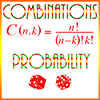# Combinations / Probability – Definitions of TermsCombinations: Groupings of a set of objects without regard to order. For example, there are 7 different combinations of the elements in the set {1, 2, 3}: {1}, {2}, {3}, {1, 2}, {1, 3}, {2, 3}, and {1, 2, 3}. Notice that in all of these the order in which they are listed is not important. Thus, for example, the set {1, 2} is exactly the same set as {2, 1}. This is in contrast to permutations, where order is important.

There are 3 combinations of these elements if we take them just 2 at a time: {1, 2}, {1, 3}, {2, 3}. Another way of saying this is that the number of combinations of 3 things, taken 2 at a time, is 3.

The number of combinations of n things taken p at a time is given by the formula

\begin{align} \frac {n!}{(n-p)!p!}\end{align}

This is normally written as

\begin{align} n \choose p\end{align}

which is said, “n choose p.”

Conditional Probability: The probability that a given event will occur if it’s known that some other event has occurred. For example, if an ace has been drawn from a pack of 52 cards, conditional probability determines the probability that another ace will be drawn.

Equally Likely Probability: If several events in a probability experiment are equally likely or probable to occur, then these events have equally likely probability. Example: The two sides of a normal coin are equally likely to turn up when the coin is tossed, and so heads and tails have the equally likely probability of 1/2.

Event: A particular set of possible outcomes of a probability experiment. Example: A rolled die showing a number less than 3. The experiment is the rolling of the die. The event is {1, 2}.

Experiment: In probability, an experiment is a procedure used to measure specific instance of outcomes of the experiment. For example, tossing a coin is an experiment, and its possible outcomes are heads and tails.

Factorial: The operation of multiplying a positive integer by every positive integer less than or equal to it. For example, 4 factorial (written 4!) is

\begin{align} 4 \times 3 \times 2 \times 1 = 24\end{align}

Impossible Probability: This is a probability of 0. If an event cannot happen, then its probability is 0. Conversely, if the probability of an event is 0, then the event cannot happen.

Independent Events: Two events are independent if the occurrence of one doesn’t affect the probability of the other. For example, if the probability that a person will be born in January is .1 and in July is .15, then if John is born in January, this doesn’t change the probability that Mary is born in July or in January or in any other month. The two events are independent, so the occurrence of one doesn’t change the probability of the occurrence of the other. Tosses of coins and rolls of dice are also independent events. If a coin toss comes up heads 20 times in a row, then the probability it will come up heads the 21st time is still 1/2, because the tosses are independent.

Outcome: A possible result of a probability experiment. Examples: In the probability experiment of tossing a coin, the possible outcomes are heads and tails. In the probability experiment of rolling a die, the possible outcomes are the numbers 1 – 6.

Permutations: Different arrangements of a set of objects, where order is important. For example, {1, 2, 3} can be ordered in 6 different ways: 123, 132, 213, 231, 312, 321. The number of permutations of a set of n objects is n! (n factorial)

Probability: A number between 0 and 1 which represents the likelihood of a certain event’s happening. It’s typically measured as a fraction, with the number of possible outcomes of the event in the denominator, and the number of favorable events in the numerator. For example, if you randomly draw a card from a pack of 52 with 4 aces in it, the probability of drawing an ace is 4/52, or 1/13.